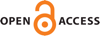Download this articleFor screen For printingRecent IssuesThe Journal About the Journal Editorial Board Editors’ Interests Subscriptions Submission Guidelines Submission Form Policies for Authors Ethics Statement ISSN: 1944-7833 (e-only) ISSN: 1937-0652 (print) Author Index To Appear Other MSP JournalsIsotriviality, integral points, and primitive primes in orbits in characteristic $p$

### Alexander Carney, Wade Hindes and Thomas J. Tucker

Vol. 17 (2023), No. 9, 1573–1594
DOI: 10.2140/ant.2023.17.1573##### Abstract

We prove a characteristic $p$ version of a theorem of Silverman on integral points in orbits over number fields and establish a primitive prime divisor theorem for polynomials in this setting. In characteristic $p$, the Thue–Siegel–Dyson–Roth theorem is false, so the proof requires new techniques from those used by Silverman. The problem is largely that isotriviality can arise in subtle ways, and we define and compare three different definitions of isotriviality for maps, sets, and curves. Using results of Favre and Rivera-Letelier on the structure of Julia sets, we prove that if $\phi$ is a nonisotrivial rational function and $\beta$ is not exceptional for $\phi$, then ${\phi }^{-n}\left(\beta \right)$ is a nonisotrivial set for all sufficiently large $n$; we then apply diophantine results of Voloch and Wang that apply for all nonisotrivial sets. When $\phi$ is a polynomial, we use the nonisotriviality of ${\phi }^{-n}\left(\beta \right)$ for large $n$ along with a partial converse to a result of Grothendieck in descent theory to deduce the nonisotriviality of the curve ${y}^{\ell }={\phi }^{n}\left(x\right)-\beta$ for large $n$ and small primes $\ell \ne p$ whenever $\beta$ is not postcritical; this enables us to prove stronger results on Zsigmondy sets. We provide some applications of these results, including a finite index theorem for arboreal representations coming from quadratic polynomials over function fields of odd characteristic.

##### Keywords
arithmetic dynamics, integral points, arboreal representations, Zsigmondy sets
##### Mathematical Subject Classification
Primary: 37P15
Secondary: 11G50, 11R32, 14G25, 37P05, 37P30
##### Milestones